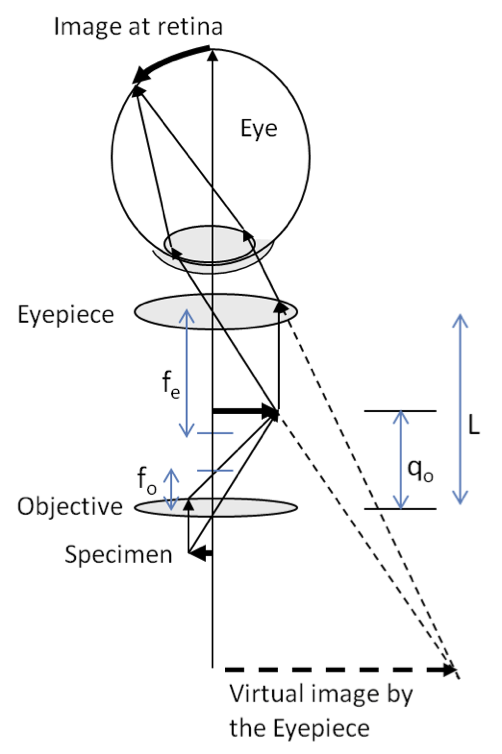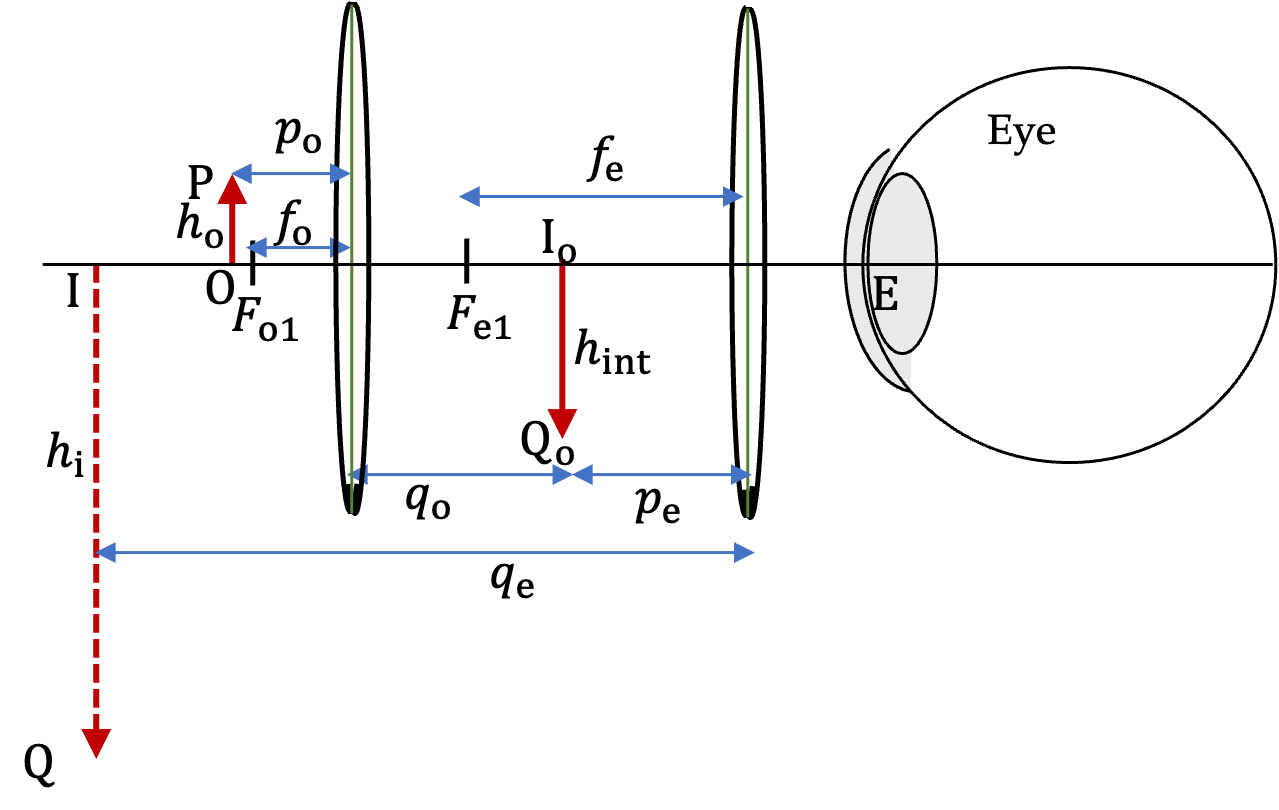## Section48.4Compound Microscope

We saw above that a simple convex lens can give magnified image when used as a magnifying glass. However, a magnification more than $5\times$ is difficult to get this way without introducing significant distortion in the image. To get larger magnification, we combine the simple magnifying glass with one or more lens. Such arrangements are called compound microscope.

The simplest compound microscope will contain two convex lenses, an objective and an eyepiece or ocular, as illustrated in Figure 48.4.1. The objective is a convex lens facing the physical object we wish to view and the eyepiece is the lens closest to the eye.

The task of the objective is to form a magnified real image at a point that is within a focal length of the eyepiece. This way, we would present a larger object for the eyepiece to further magnify in the same way a magnifying glass works as explained in Section 48.3.

Hence, we place the specimen a little bit outside of the first focal point of the objecive and arrange the lenses so that a magnified real image by the objective lens is formed inside the focal length of the eyepiece. The image by the eyepiece is therefore a magnified virtual image for eye to look at.Figure 48.4.1. Compound microscope. The specimen forms a magnified real image at distance $q_0$ from the objective. This real image is object for the eyepiece, which forms magnified virtual image. This virtual image forms object for the eye, which forms a real image at the retina.

### Subsection48.4.1Magnification of a Compound Microscope

Figure 48.4.2 shows image formation by the two lenses and various distances involved. The object, i.e., the speciment is at OP of height $h_\text{o}\text{.}$ The objective forms a real image of height $h_\text{int}$ at $\text{I}_\text{o}\text{Q}_\text{o}$ with magnification $m_\text{obj}$ given by

\begin{equation*} m_\text{obj} = \frac{h_\text{int}}{h_\text{o}} = -\frac{q_\text{o}}{p_\text{o}}. \end{equation*}

Usually, for max magnification from the objective, the speciment is very small distance greater than the focal length of the objective, $f_\text{o}\text{.}$

\begin{equation*} m_\text{obj} \approx -\frac{q_\text{o}}{f_\text{o}}. \end{equation*}

This number is usually referred to some number X on the objective of the microscope. For instance, $50\text{X}$ objective means you have $m_\text{obj} = 50\text{.}$

We now look at this real image $\text{I}_\text{o}\text{Q}_\text{o}$ using the eyepiece as a magnifying glass. Let $n_p$ denote the distance to the near point of the eye. The magnification of the magnifying glass was done by angular magnification.

\begin{equation*} M_\text{eyepiece/eye} = 1 + \frac{n_p}{f_e}. \end{equation*}

This number is usually referred to some number X on the eyepiece of the microscope. For instance, $10\text{X}$ eyepiece means you have $M_\text{eyepiece/eye}=10\text{.}$

Multiplying these two sources of magnification gives us the magnification of the microscope, which will be the angular magnification.

\begin{equation} M_\text{microscope} = m_\text{obj} \times M_\text{eyepiece/eye} = -\frac{q_\text{o}\left(f_\text{e} + n_p \right)}{ f_\text{o} f_\text{e}}.\label{eq-compound-microscope-magnification-formula}\tag{48.4.1} \end{equation}

Thus, if you have a $50\text{X}$ objective and a $10\text{X}$ eyepiece, you will get a microscope that has $500\text{X}$ magnification. The magnification of the microscope is sometimes referred to as the magnifying power.

We can multiply the size of the specimen $h_\text{o}$ with this $M$ of the microscope to get the apparent size of the image perceived.

\begin{equation*} \text{Height Perceived} = M_\text{microscope}\ h_\text{o}. \end{equation*}Figure 48.4.2. Optical parameters for image formation and magnification calculations of a compound microscope.

The objective and eyepiece of a compound microscope have focal lengths $2.5\text{ cm}$ and $10.0\text{ cm}$ respectively. You use this microscope to observe a $70\, \mu\text{m}$ specimen placed $3.0\text{ cm}$ from the objective. Assuming your near point is $25\text{ cm}$ from your eye, how large is would be the image you would perceive?

Hint

Work out the magnification first. Since $p_\text{o}\ne f_\text{o}\text{,}$ its best to find magnification of the objective by using $m=-q/p\text{.}$

$1.23\text{ mm}\text{.}$

Solution

We need to find $q_0$ to find the magnification. We do that from the lens equation applied to the objective.

\begin{align*} q_\text{o} \amp = \frac{p_\text{o}\, f_\text{o}}{p_\text{o} - f_\text{o}} \\ \amp = \frac{3 \times 2.5}{3- 2.5} = 15\text{ cm}. \end{align*}

This gives objective to be magnifying by a factor of

\begin{equation*} m_\text{obj} = - q_\text{o}/p_\text{o} = -5. \end{equation*}

That is the objective is $5\times\text{.}$ The magnification of the eyepiece/eye is

\begin{equation*} M_\text{eyepiece} = 1 + \frac{n_p}{f_\text{e}} = 1 + \frac{25\text{ cm}}{10\text{ cm}} = 3.5. \end{equation*}

That is the eyepiece is $3.5\times\text{.}$ Now, we multiply the two to get Therefore,

\begin{align*} M_\text{microscope} \amp = -5\times 3.5 = -17.5. \end{align*}

Therefore, the size perceived will be $70\times 17.5$ $= 1225\,\mu\text{m}$ $= 1.23\text{ mm}\text{.}$

A hair is viewed in a microscope with a $20\text{X}$ object and a $5\text{X}$ eyepiece. The hair appears to to be $5\text{ mm}$ thick. What is the actual thickness of the hair.

Hint

Divide by the magnification of the microscope.

$0.05\text{ mm}\text{.}$